# How to solve for unknown charge position

• PhizKid
In summary, the student attempted to solve for the location of an unknown charge by considering how two forces would completely cancel each other. They drew two possible solutions and were not sure what to do from there. They needed to find the relative directions of the forces and found that they must be perpendicular to each other. Additionally, they needed to find the directions of the electric forces between the fixed charges and the unknown charge. Once they had these information, they were able to narrow the search down to one region.f

## Homework Statement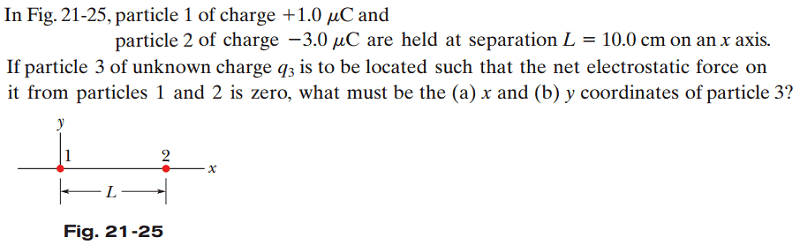## Homework Equations

Coulomb's Law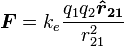## The Attempt at a Solution

Here are 2 different possibilities I drew that I think will have the x component vectors and y component vectors cancel out, but I think there may be some other positions as well.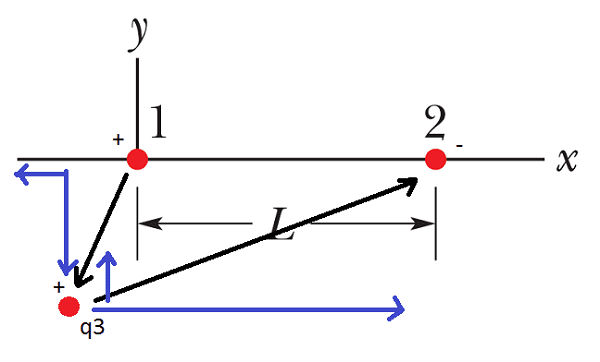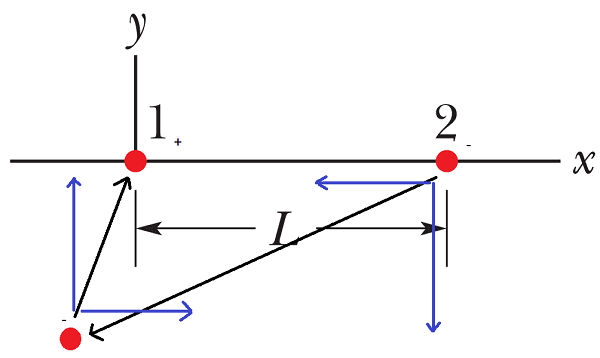##\textbf{F}_x = k_e [\frac{(1.0 \textrm{ uC} \cdot q3) \cdot cos(\theta_1) \cdot \hat{r}_{13}}{{r_1}^2} + \frac{(-3.0 \textrm{ uC} \cdot q3) \cdot cos(\theta_2) \cdot \hat{r}_{23}}{{r_2}^2}]##

##\textbf{F}_y = k_e [\frac{(1.0 \textrm{ uC} \cdot q3) \cdot sin(\theta_1) \cdot \hat{r}_{13}}{{r_1}^2} + \frac{(-3.0 \textrm{ uC} \cdot q3) \cdot sin(\theta_2) \cdot \hat{r}_{23}}{{r_2}^2}]##

But there are so many unknowns and I only have one equation (Coulomb's) so I'm not sure what to do from here.

You should be able to narrow the search considerably by considering:

1) If two forces are to completely cancel, what must be their relative directions?
2) What are the directions that the electric forces act between the fixed charges and the unknown charges?

In what way do the answers to the above two items constrain the possible location of q3?

Hint: For two vectors to be able to cancel each other, what angle must they make with each other?

Edit: gneill beat me to it!

You should be able to narrow the search considerably by considering:

1) If two forces are to completely cancel, what must be their relative directions?
2) What are the directions that the electric forces act between the fixed charges and the unknown charges?

Oh, as I was thinking about answering your questions I just realized I need to completely review vectors...but now I see that the unknown charge has to be somewhere axis along the fixed charges, but since the force is inversely proportional to the distance, it can't be on the right side because the charge on the right would overpower the one on the left.

Okay, so the magnitudes of the two forces have to equal each other:

##k_e \cdot \frac{|q_1||q_3|}{{r_1}^2} = k_e \cdot \frac{|q_2||q_3|}{({r_1 + L)}^2}##

Cancel out the constant and unknown charge:

##\frac{|q_1|}{{r_1}^2} = \frac{|q_2|}{({r_1 + L)}^2}##

But here I have a question about the signs of the force. Since we're only working with the magnitude, do I need to add a negative sign somewhere?

I suggest that you make another drawing and use it to further narrow down the region of interest. Then you can write expressions for the force magnitudes without any signs involved. For example: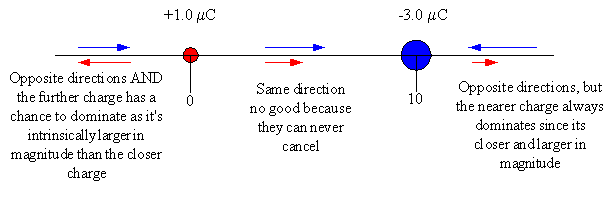The diagram shows there's only one region that the third charge can be located so that zero net force can occur.

Since all the given values have similar units (μC, cm), you can drop those too when you write the equality.

#### Attachments

•1 person